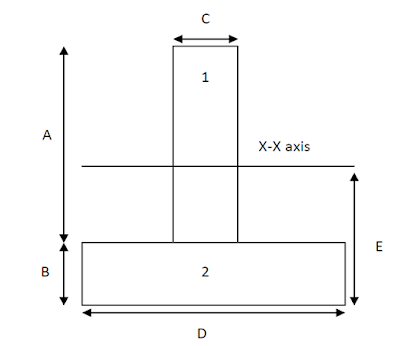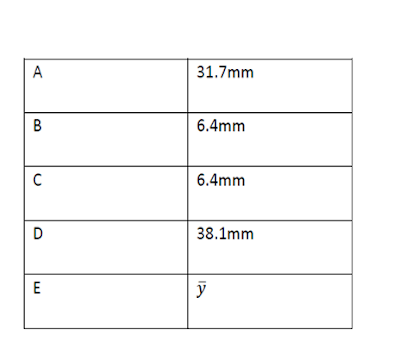# Bending Stresses in Beam Lab ReportAim
Aim of this experiment is to study the effect of force of different magnitude on the bending stresses in beam

## Recommended: Deflection of Beam Lab Report (Simply Supported Beam)

Hire Us for your Lab Report Writing
Theory
Bending of Beam
When an external load or the structural load applied in beam is large enough to displace the beam from its present place, then that deflection of beam from its resent axis is called bending of beam.

Bending stresses
That applied force which produce bending in beam also produces stresses in beam these stresses are called bending stresses.

Introduction to Experiment
This experiment is about studying the effect of force of different magnitude on the bending stresses in a beam. To perform this experiment a structural hardware ‘STR5 Bending stress in a beam” will be used. Following is the figure of that apparatusA T shape beam is being used in this experiment which has been supported on the apparatus with the help of two simple pivots. In this experiment load is applied on two different places with the help of special mechanism attached to the apparatus. There are strain gauges attached to the beam at different locations and these strain gauges are used to collect the data of stress produce in the beam. Following is the free body diagram of the beam attached to the apparatus and under the load at two different points.Bending stresses are produce in a beam when an external force is applied on the beam and produce deflection in the beam. Bending stresses main depends on the shape of beam, length of beam and magnitude of the force applied on the beam. In order to calculate the bending stresses in the beam following formula can be used

E = σ/ε

M/I  ×σ/y

Here
E is the young modulus
M is the bending moment
I is the second moment of inertia of beam
σ is the bending stress (Nm-1)
ε  is the bending Strain
Y is the distance from the neutral axis

Procedure
The apparatus involve in this experiment use an electronic system intrigued with software to apply load and calculate the bending moment. Due to which it is very important to follow the steps involve in this experiment in following presented order
• Set up the computer software using the guide provided with the apparatus and set it to virtual experiment mode
• In property section box, select the option of variable hanger load
• From the tool box area take a load of 100 g and replace it with 0 gram at the cut section
• Diagram of the force applied and the graph of the resultant force will appear of screen which conform the load replacement.
• Software will automatically gather all the data of experiment and save it in memory.
• Repeat the third step with 200, 300, 400 and 500 grams and collect the data related to each experiment
• Final result provided by software and manually calculation made were compared using graphs

## Results

Table 5  bending stresses results 1
 Gauge numbers Vertical position (nominal) Vertical position (actual) Ave strains where bending moment is: Ave strains where bending moment is: Ave strains where bending moment is: Ave strains where bending moment is: Ave strains where bending moment is: Ave strains where bending moment is: Ave strains where bending moment is: Ave strains where bending moment is: Ave strains where bending moment is: Ave strains where bending moment is: mm mm 0.1 Nm 17.7 Nm 17.5 Nm 35.1 Nm 35.1 Nm 53.0 Nm 52.7 Nm 52.4 Nm 70.2 Nm 75.0 Nm 1 0.0 ??? -27.8 -28.1 -27.6 -28.0 -27.9 -27.7 -28.1 -27.9 -28.2 -27.8 2,3 8.0 ??? -54.2 -54.7 -54.2 -54.4 -54.4 -54.0 -54.5 -54.3 -54.4 -54.3 4,5 23.0 ??? -36.6 -37.0 -36.6 -36.6 -36.8 -36.2 -36.8 -36.6 -36.5 -36.6 6,7 31.7 ??? -33.1 -33.6 -33.2 -33.2 -33.4 -32.9 -33.4 -33.0 -33.2 -33.3 8,9 38.1 ??? -30.9 -31.3 -31.2 -31.0 -31.1 -30.9 -31.2 -30.9 -31.0 -31.0

Table 6  bending stresses results 2
 Load Bending Moment Gauge 1 Strain Gauge 2 Strain Gauge 3 Strain Gauge 4 Strain Gauge 5 Strain Gauge 6 Strain Gauge 7 Strain Gauge 8 Strain Gauge 9 Strain Gauge 10 Strain N Nm           0.4 0.1 -27.8 -81.8 -26.7 -46.5 -26.6 -45.6 -20.7 -46.3 -15.4 -- 100.2 17.5 -27.6 -81.8 -26.6 -46.5 -26.6 -45.9 -20.5 -46.9 -15.5 -- 200.5 35.1 -27.9 -82.0 -26.8 -46.9 -26.8 -46.0 -20.8 -46.7 -15.6 -- 299.7 52.4 -27.9 -82.0 -26.7 -46.7 -26.6 -45.5 -20.5 -46.6 -15.3 -- 401.3 70.2 -28.2 -82.1 -26.7 -46.4 -26.7 -45.7 -20.7 -46.6 -15.3 -- 428.3 75.0 -27.8 -82.0 -26.6 -46.7 -26.5 -45.9 -20.8 -46.5 -15.5 --

Calculations

According to the equation shown above for calculating bending stresses and strain, there are certain quantities like area of cross section of beam sections and second moment of inertia of beam needed to be known. Following are the equation which will be used for solving these quantities.y̅* Total area = first Area and its distance from the X-X axis + second area and its distance from X-X axis

First Area = 31.7 × 6.4 = 202.88 mm^2

Second Area = 38.1 ×6.4 = 243.84 mm^2

Total Area = 202.88 +243.84 = 446.72 mm^2

y̅* 446.72 = 446.72 * 22.25 + 243.84 * 3.2

y̅=  (202.88 × 22.25+243.84 × 3.2 )/446.72

y̅= 11.85 × 〖10〗^(-3)  m.

Beam height = 11.85 + 26.25 = 38.1 mm

Second moment of area along x-x axis

IXX = A*h2 + IXX base

IXX = (Area) × (y̅ -y2)2 + (bd^3)/12

IXX= (6.4×38.1) × (11.85 – 3.2)2 + (6.4  ×  〖31.7〗^3)/12 + (6.4× 31.7) × (11.85 – 22.25)2 + (38.1 ×  〖6.4〗^3)/12

IXX = 58009mm4

Bending stresses

σ =  (M.y)/I

Theoretical

σ =  (17.5 *  11.85*〖10〗^(-3))/(58*10^(-9) )=3.5*〖10〗^6  N/m

Experimental
σ =  (17.5 ×26.25 × 〖10〗^(-3))/(58.009 × 〖10〗^(-9) ) = 7.9 * 〖10〗^6  N/m

 Load Bending moment Experimental bending Stress Theoretical Bending Stress N Nm Nm Nm 0.4 0.1 0.0450.0204100.2 17.5 7.93.6200.5 35.1 15.8387.17299.7 52.4 23.75710.70401.3 70.2 31.72114.34428.3 75.0 33.9115.32## Discussion

Values of the bending stresses obtain from the experiment are presented in the table above and they are arranged in the respective cell according to the load that produce that bending stresses. All the data is presented in the graphs and according to that graph the experimental bending stress is showing linear relation with the load means the value of the experimental bending stresses increase with the increase in the value of applied load and decrease with the decrease in the value of applied load. The ratio with which there is an increase and decrease in the value of experimental bending stresses is equal to the ratio with which there is an increase and decrease in the value of applied load.

According to the first graph the relationship between the theoretical bending stress and the applied load which looks like linear just like the experimental bending stress but in this relation the value of the theoretical bending stress does not change as much as the value of experimental bending stress change with respect of change in applied load. There is greater increase in the value of load than the increase in the value of the theoretical bending stress

## Conclusion

Aim of this task was to study the effect of different forces on the bending stresses in the beam and the result show that there is a linear relationship between bending stress and applied load. Experimental bending stress show perfect linear relationship with applied load whereas the theoretical bending stress does not respond that much in increase of applied load.

1.2.